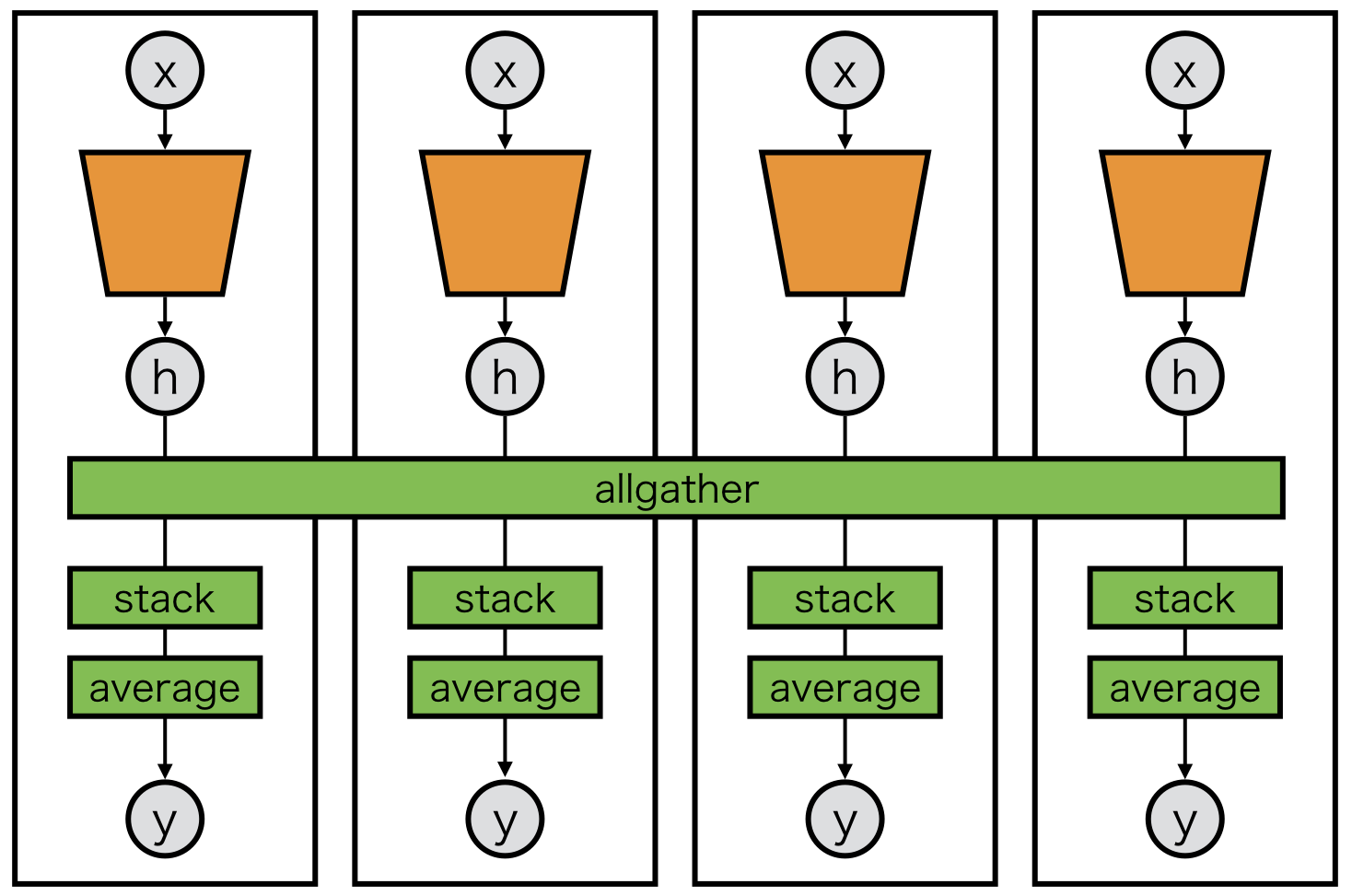# Example 4: Ensemble¶

Ensemble is a training technique to obtain better classification performance by combining multiple base classifiers. Averaging ensemble is one of the simplest examples of ensemble, which takes average of all classifier outputs in the test phase. Model parallelism and collective communications can effectively help to implement it.The following wrapper makes model parallel averaging ensemble easier:

class Averaging(chainer.Chain):
def __init__(self, comm, block):
super(Averaging, self).__init__()
self.comm = comm
with self.init_scope():
self.block = block

def __call__(self, x):
y = self.block(x)

if not chainer.config.train:
y = chainermn.functions.allgather(self.comm, y)
y = F.stack(y, axis=0)
y = F.average(y, axis=0)

return y


Then, any links wrapped by Averaging are ready to be parallelized and averaged:

class Model(chainer.Chain):
def __init__(self, comm):
super(Model, self).__init__()
self.comm = comm
with self.init_scope():
self.l1 = L.Linear(d0, d1)
self.l2 = L.Linear(d1, d2)
self.l3 = Averaging(self.comm, L.Linear(d2, d3))

def __call__(self, x):
h = F.relu(self.l1(x))
h = F.relu(self.l2(h))
y = F.relu(self.l3(h))
return y


From the perspective of model inputs/outputs, the averaged model is compatible with the original model. Thus, we only need to replace the last layer with the averaged layer.

In averaging ensemble, each base classifier is trained independently and ensembled in the test phase. This can be implemented by using MultiNodeIterator only for the test iterator:

# train = (training dataset)
# test = (test dataset)

if comm.rank != 0:
train = chainermn.datasets.create_empty_dataset(train)
test = chainermn.datasets.create_empty_dataset(test)

train_iter = chainer.iterators.SerialIterator(train, batchsize)
test_iter = chainermn.iterators.create_multi_node_iterator(
chainer.iterators.SerialIterator(test, batchsize,
repeat=False, shuffle=False),
comm)